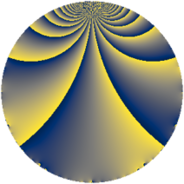# Properties

 Label 441.1.bLevel $441$ Weight $1$ Character orbit 441.b Rep. character $\chi_{441}(197,\cdot)$ Character field $\Q$ Dimension $2$ Newform subspaces $1$ Sturm bound $56$ Trace bound $0$

# Related objects

## Defining parameters

 Level: $$N$$ $$=$$ $$441 = 3^{2} \cdot 7^{2}$$ Weight: $$k$$ $$=$$ $$1$$ Character orbit: $$[\chi]$$ $$=$$ 441.b (of order $$2$$ and degree $$1$$) Character conductor: $$\operatorname{cond}(\chi)$$ $$=$$ $$3$$ Character field: $$\Q$$ Newform subspaces: $$1$$ Sturm bound: $$56$$ Trace bound: $$0$$

## Dimensions

The following table gives the dimensions of various subspaces of $$M_{1}(441, [\chi])$$.

Total New Old
Modular forms 18 2 16
Cusp forms 2 2 0
Eisenstein series 16 0 16

The following table gives the dimensions of subspaces with specified projective image type.

$$D_n$$ $$A_4$$ $$S_4$$ $$A_5$$
Dimension 2 0 0 0

## Trace form

 $$2q - 2q^{4} + O(q^{10})$$ $$2q - 2q^{4} - 2q^{16} - 4q^{22} + 2q^{25} + 4q^{46} + 4q^{58} + 2q^{64} - 4q^{67} + 4q^{79} + O(q^{100})$$

## Decomposition of $$S_{1}^{\mathrm{new}}(441, [\chi])$$ into newform subspaces

Label Dim. $$A$$ Field Image CM RM Traces $q$-expansion
$$a_2$$ $$a_3$$ $$a_5$$ $$a_7$$
441.1.b.a $$2$$ $$0.220$$ $$\Q(\sqrt{-2})$$ $$D_{4}$$ $$\Q(\sqrt{-7})$$ None $$0$$ $$0$$ $$0$$ $$0$$ $$q-\beta q^{2}-q^{4}-\beta q^{11}-q^{16}-2q^{22}+\cdots$$